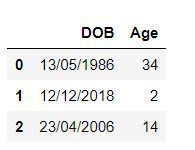# Convert birth date to age in Pandas

• Last Updated : 02 Feb, 2021

In this article, we are going to convert birthdate into the age in pandas dataframe. We will be using Pandas and datetime package to convert birth date into age. To convert the date of birth to age first we convert the given date to the right format by using strptime() function and then subtract current year with birthdate year and check if the birth month and birth date are greater than a current month and current date if it is true we subtract one else zero is subtracted.

Approach:

• First, we use strptime function to identify the given date format into the date, month, and year.
• Then we use today function to get today’s date.
• To get age we subtract the birth year from the current year. This gives age in years but, to calculate accurate age further we check if the birth month and birth date is greater than current month and the current date and if this condition is true we subtract 1 from the final result as even if the current year is passed but yet his birth month or birth date is still yet to arrive.

Example 1: In this example, we will convert a single given date to age.

## Python3

 `from` `datetime ``import` `datetime, date`` ` `born``=``'26/01/2000'``print``(``"Born :"``,born)`` ` `#Identify given date as date month and year``born ``=` `datetime.strptime(born, ``"%d/%m/%Y"``).date()`` ` `#Get today's date``today ``=` `date.today()`` ` `print``(``"Age :"``,``      ``today.year ``-` `born.year ``-` `((today.month,``                                          ``today.day) < (born.month,``                                                        ``born.day)))`

Output:

```Born : 26/01/2000
Age : 21```

Explanation: In the above code, we used datetime package and imported datetime and time. We used strptime function to identify date stored in the born variable i.e. we identified 26/01/2000 as date/month/year. Then we used today() function to get today’s date. To get age we used formula today.year – born.year – ((today.month, today.day) < (born.month, born.day). In this, we subtract a current year from the born year, and then if the current date and month have not passed born date and month we subtract one as his/her birth date and month is yet to come.

Example 2: Now we will use a dataframe having a column of date of birth and convert it to age and add that column to that dataframe.

## Python3

 `import` `pandas as pd``from` `datetime ``import` `datetime, date`` ` `# Creating a list of date of birth``dob ``=` `{``'DOB'``: [``'13/05/1986'``, ``'12/12/2018'``, ``'23/04/2006'``]}`` ` `# Creating dataframe``df ``=` `pd.DataFrame(data ``=` `dob)`` ` `# This function converts given date to age``def` `age(born):``    ``born ``=` `datetime.strptime(born, ``"%d/%m/%Y"``).date()``    ``today ``=` `date.today()``    ``return` `today.year ``-` `born.year ``-` `((today.month, ``                                      ``today.day) < (born.month, ``                                                    ``born.day))`` ` `df[``'Age'``] ``=` `df[``'DOB'``].``apply``(age)`` ` `display(df)`

Output:Explanation: In above code used pandas and datetime package. We created a dataframe of DOB having three rows of different dates. To calculate age we created an age function that uses strptime function to identify the date in date/month/year format. Then we used today() function to get today’s date. To get age we used formula today.year – born.year – ((today.month, today.day) < (born.month, born.day). In this, we subtract a current year from the born year, and then if the current date and month have not passed born date and month we subtract one as his/her birth date and month is yet to come. We return age in this function which gets added as a new row inside column ‘Age’. Later we display the data frame.

My Personal Notes arrow_drop_up